International Tables for Crystallography (2006). Vol. D, ch. 2.1, pp. 266-293https://doi.org/10.1107/97809553602060000638

## Contents

• 2.1. Phonons  (pp. 266-293)
• 2.1.1. Introduction  (p. 266) | html | pdf |
• 2.1.2. Fundamentals of lattice dynamics in the harmonic approximation  (pp. 266-274) | html | pdf |
• 2.1.2.1. Hamiltonian and equations of motion  (pp. 266-267) | html | pdf |
• 2.1.2.2. Stability conditions  (p. 267) | html | pdf |
• 2.1.2.3. The dynamical matrix  (pp. 267-268) | html | pdf |
• 2.1.2.4. Eigenvalues and phonon dispersion, acoustic modes  (pp. 268-270) | html | pdf |
• 2.1.2.5. Eigenvectors and normal coordinates  (p. 270) | html | pdf |
• 2.1.2.6. Amplitudes of lattice vibrations  (pp. 270-271) | html | pdf |
• 2.1.2.7. Density of states and the lattice heat capacity  (pp. 271-272) | html | pdf |
• 2.1.2.8. Thermal expansion, compressibility and Grüneisen parameters  (pp. 272-274) | html | pdf |
• 2.1.3. Symmetry of lattice vibrations  (pp. 274-291) | html | pdf |
• 2.1.3.1. Symmetry constraints for the dynamical matrix  (pp. 274-281) | html | pdf |
• 2.1.3.1.1. Example  (pp. 277-281) | html | pdf |
• 2.1.3.2. Symmetry of dispersion planes  (p. 281) | html | pdf |
• 2.1.3.3. Symmetry properties of eigenvectors  (pp. 281-283) | html | pdf |
• 2.1.3.3.1. Example  (p. 283) | html | pdf |
• 2.1.3.4. Symmetry coordinates  (pp. 283-286) | html | pdf |
• 2.1.3.4.1. Example  (pp. 284-286) | html | pdf |
• 2.1.3.5. Degeneracy of lattice vibrations  (pp. 286-289) | html | pdf |
• 2.1.3.5.1. Accidental degeneracy  (p. 286) | html | pdf |
• 2.1.3.5.2. Time-reversal degeneracy  (pp. 286-288) | html | pdf |
• 2.1.3.5.3. Example  (pp. 288-289) | html | pdf |
• 2.1.3.6. Compatibility relations  (pp. 289-290) | html | pdf |
• 2.1.3.6.1. Example  (pp. 289-290) | html | pdf |
• 2.1.3.7. Optical selection rules  (pp. 290-291) | html | pdf |
• 2.1.3.7.1. Example  (p. 291) | html | pdf |
• 2.1.4. Conclusion  (p. 291) | html | pdf |
• 2.1.5. Glossary  (pp. 291-292) | html | pdf |
• References | html | pdf |
• Figures
• Fig. 2.1.2.1. Definition of position vectors  (p. 266) | html | pdf |
• Fig. 2.1.2.2. Definition of the force acting on atom (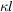) when atom (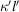) is displaced by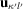(p. 267) | html | pdf |
• Fig. 2.1.2.3. Phonon dispersion of b.c.c. hafnium for wavevectors along the main symmetry directions of the cubic structure  (p. 268) | html | pdf |
• Fig. 2.1.2.4. Phonon dispersion of Nd 2 CuO 4 along the main symmetry directions of the tetragonal structure  (p. 269) | html | pdf |
• Fig. 2.1.2.5. Low-frequency part of the phonon dispersion of deuterated naphthalene at 6 K  (p. 269) | html | pdf |
• Fig. 2.1.2.6. Energy levels of a quantum-mechanical harmonic oscillator  (p. 271) | html | pdf |
• Fig. 2.1.2.7. Phonon dispersion and density of states for GaAs  (p. 271) | html | pdf |
• Fig. 2.1.2.8. Schematic representation of the true phonon density of states (solid line) along with the Debye approximation (dotted line)  (p. 272) | html | pdf |
• Fig. 2.1.2.9. Temperature dependence of the heat capacity at constant volume according to the Debye model  (p. 272) | html | pdf |
• Fig. 2.1.3.1. Transformation of atomic displacements by a symmetry operation  (p. 275) | html | pdf |
• Fig. 2.1.3.2. Relation between interaction of symmetry-related atoms  (p. 275) | html | pdf |
• Fig. 2.1.3.3. Symmetry-related atoms in different primitive cells  (p. 276) | html | pdf |
• Fig. 2.1.3.4. Projection along the tetragonal z axis of the example structure given in Table 2.1.3.1  (p. 278) | html | pdf |
• Fig. 2.1.3.5. Symmetry of the dispersion surface  (p. 281) | html | pdf |
• Fig. 2.1.3.6. The dispersion relation is an even function of q   (p. 281) | html | pdf |
• Fig. 2.1.3.7. Matrix of symmetry coordinates at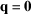for the example structure given in Fig. 2.1.3.4 and Table 2.1.3.1  (p. 287) | html | pdf |
• Fig. 2.1.3.8. ( a ) Accidental degeneracy of phonons with different symmetry  (p. 287) | html | pdf |
• Fig. 2.1.3.9. Low-frequency part of the phonon dispersion of KLiSO 4 at room temperature (space group P 6 3 )  (p. 289) | html | pdf |
• Fig. 2.1.3.10. Illustration of the compatibility relations for phonons in a tetragonal crystal with space groupfor wavevectors along [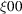]  (p. 290) | html | pdf |
• Fig. 2.1.3.11. Principle of infrared absorption  (p. 290) | html | pdf |
• Fig. 2.1.3.12. Principle of Raman spectroscopy  (p. 290) | html | pdf |
• Tables
• Table 2.1.3.1. Example structure in space group(p. 278) | html | pdf |
• Table 2.1.3.2. Atom transformation table  (p. 278) | html | pdf |
• Table 2.1.3.3. Character table of the point group(p. 283) | html | pdf |
• Table 2.1.3.4. Irreducible representations of the point group(p. 288) | html | pdf |
• Table 2.1.3.5. Irreducible representations of the space groupfor(thepoint)  (p. 289) | html | pdf |
• Table 2.1.3.6. Irreducible representations of the space group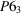for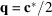(the A point)  (p. 289) | html | pdf |
• Table 2.1.3.7. Irreducible representations of the space groupfor(thepoint)  (p. 290) | html | pdf |
• Table 2.1.3.8. Character table of the space groupfor(thepoint)  (p. 291) | html | pdf |